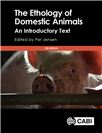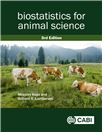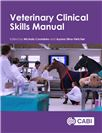Like most websites we use cookies. This is to ensure that we give you the best experience possible.

CAB eBooks

Ebooks on agriculture and the applied life sciences from CAB International

Results per page:

Search results

CABI Book Chapter InfoThe study of animal behaviour and its applications.

This chapter provides a brief overview of the historical background and of the various fields into which ethology has branched over the decades. The field that is of most interest in this book is the application of ethology in the study of animals utilized by man.

Author(s)
Jensen, P.
ISBN
2017 CABI (H ISBN 9781786391650)
Type
Book chapter
CABI Book Chapter InfoCurvilinear regression.

This chapter describes the use of curvilinear regression analysis to determine the non-linear effects of an independent variable on a dependent variable.

Author(s)
Kaps, M.; Lamberson, W. R.
ISBN
2017 CABI (H ISBN 9781786390356)
Type
Book chapter
CABI Book Chapter InfoRandom effects one-way analysis of variance.

This chapter describes the differences between fixed and random effects of one-way analysis of variance and the application of random effects analysis of variance in animal production studies.

Author(s)
Kaps, M.; Lamberson, W. R.
ISBN
2017 CABI (H ISBN 9781786390356)
Type
Book chapter
CABI Book Chapter InfoDiscrete, categorical and other non-normal dependent variables.

The functions of discrete, categorical and non-normal dependent variables in linear models with both dependent and independent variables are discussed.

Author(s)
Kaps, M.; Lamberson, W. R.
ISBN
2017 CABI (H ISBN 9781786390356)
Type
Book chapter
CABI Book Chapter InfoPresenting and summarizing data.

This chapter discusses the collection and summarization of experimental data in animal production studies prior to statistical analysis.

Author(s)
Kaps, M.; Lamberson, W. R.
ISBN
2017 CABI (H ISBN 9781786390356)
Type
Book chapter
CABI Book Chapter InfoEstimation of parameters.

The concept of parameter estimation in statistical analysis and its application in animal production studies is discussed.

Author(s)
Kaps, M.; Lamberson, W. R.
ISBN
2017 CABI (H ISBN 9781786390356)
Type
Book chapter
CABI Book Chapter InfoHypothesis testing.

This chapter discusses the use of probability rules to test null and alternative hypotheses in statistical analysis.

Author(s)
Kaps, M.; Lamberson, W. R.
ISBN
2017 CABI (H ISBN 9781786390356)
Type
Book chapter
CABI Book Chapter InfoSimple linear regression.

This chapter discusses the use of linear regression analysis to determine how changes in one variable can affect another variable and its application in animal production studies.

Author(s)
Kaps, M.; Lamberson, W. R.
ISBN
2017 CABI (H ISBN 9781786390356)
Type
Book chapter
CABI Book Chapter InfoCorrelation.

The concept of correlation analysis to determine the relationship between two statistical variables is described.

Author(s)
Kaps, M.; Lamberson, W. R.
ISBN
2017 CABI (H ISBN 9781786390356)
Type
Book chapter
CABI Book Chapter InfoUrine and faecal analysis.

This chapter discussed the techniques in urinalysis for initial screening using specific gravity measurement and dipstick. Also highlighted are methods of preparing urine sample for examination under the microscope and the use of McMaster faecal egg count technique for gastrointestinal parasites.

Author(s)
Langridge, A.; English, K.
ISBN
2018 CAB International (H ISBN 9781786391629)
Type
Book chapter

More results…

More results…

More results…